This book is archived and will be removed July 6, 2022. Please use the updated version.

Sources of Magnetic Fields

# 77 The Biot-Savart Law

### Learning Objectives

By the end of this section, you will be able to:

• Explain how to derive a magnetic field from an arbitrary current in a line segment
• Calculate magnetic field from the Biot-Savart law in specific geometries, such as a current in a line and a current in a circular arc

We have seen that mass produces a gravitational field and also interacts with that field. Charge produces an electric field and also interacts with that field. Since moving charge (that is, current) interacts with a magnetic field, we might expect that it also creates that field—and it does.

The equation used to calculate the magnetic field produced by a current is known as the Biot-Savart law. It is an empirical law named in honor of two scientists who investigated the interaction between a straight, current-carrying wire and a permanent magnet. This law enables us to calculate the magnitude and direction of the magnetic field produced by a current in a wire. The Biot-Savart law states that at any point P ((Figure)), the magnetic field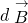due to an element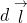of a current-carrying wire is given by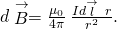A current element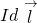produces a magnetic field at point P given by the Biot-Savart law.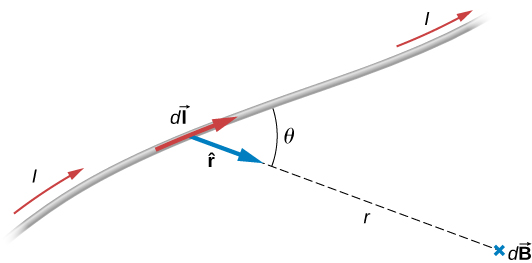The constant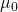is known as the permeability of free space and is exactly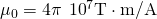in the SI system. The infinitesimal wire segmentis in the same direction as the current I (assumed positive), r is the distance fromto P and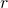is a unit vector that points fromto P, as shown in the figure.

The direction ofis determined by applying the right-hand rule to the vector product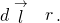The magnitude ofis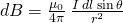where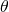is the angle betweenand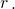Notice that if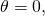then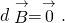The field produced by a current elementhas no component parallel to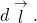The magnetic field due to a finite length of current-carrying wire is found by integrating (Figure) along the wire, giving us the usual form of the Biot-Savart law.

Biot-Savart law

The magnetic field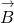due to an elementof a current-carrying wire is given by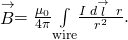Since this is a vector integral, contributions from different current elements may not point in the same direction. Consequently, the integral is often difficult to evaluate, even for fairly simple geometries. The following strategy may be helpful.

Problem-Solving Strategy: Solving Biot-Savart Problems

To solve Biot-Savart law problems, the following steps are helpful:

1. Identify that the Biot-Savart law is the chosen method to solve the given problem. If there is symmetry in the problem comparingand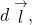Ampère’s law may be the preferred method to solve the question.
2. Draw the current element lengthand the unit vector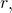noting thatpoints in the direction of the current andpoints from the current element toward the point where the field is desired.
3. Calculate the cross productThe resultant vector gives the direction of the magnetic field according to the Biot-Savart law.
4. Use (Figure) and substitute all given quantities into the expression to solve for the magnetic field. Note all variables that remain constant over the entire length of the wire may be factored out of the integration.
5. Use the right-hand rule to verify the direction of the magnetic field produced from the current or to write down the direction of the magnetic field if only the magnitude was solved for in the previous part.

Calculating Magnetic Fields of Short Current Segments A short wire of length 1.0 cm carries a current of 2.0 A in the vertical direction ((Figure)). The rest of the wire is shielded so it does not add to the magnetic field produced by the wire. Calculate the magnetic field at point P, which is 1 meter from the wire in the x-direction.

A small line segment carries a current I in the vertical direction. What is the magnetic field at a distance x from the segment?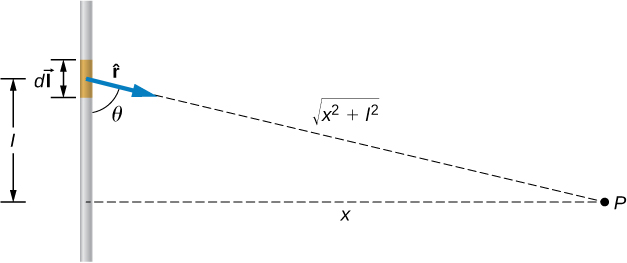Strategy We can determine the magnetic field at point P using the Biot-Savart law. Since the current segment is much smaller than the distance x, we can drop the integral from the expression. The integration is converted back into a summation, but only for small dl, which we now write as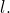Another way to think about it is that each of the radius values is nearly the same, no matter where the current element is on the line segment, ifis small compared to x. The angleis calculated using a tangent function. Using the numbers given, we can calculate the magnetic field at P.

Solution The angle between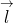andis calculated from trigonometry, knowing the distances l and x from the problem: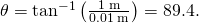The magnetic field at point P is calculated by the Biot-Savart law: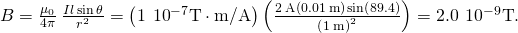From the right-hand rule and the Biot-Savart law, the field is directed into the page.

Significance This approximation is only good if the length of the line segment is very small compared to the distance from the current element to the point. If not, the integral form of the Biot-Savart law must be used over the entire line segment to calculate the magnetic field.

Check Your Understanding Using (Figure), at what distance would P have to be to measure a magnetic field half of the given answer?

1.41 meters

Calculating Magnetic Field of a Circular Arc of Wire A wire carries a current I in a circular arc with radius R swept through an arbitrary angle((Figure)). Calculate the magnetic field at the center of this arc at point P.

A wire segment carrying a current I. The pathand radial directionare indicated.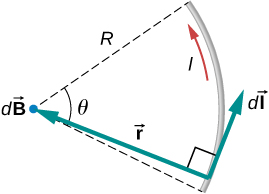Strategy We can determine the magnetic field at point P using the Biot-Savart law. The radial and path length directions are always at a right angle, so the cross product turns into multiplication. We also know that the distance along the path dl is related to the radius times the angle(in radians). Then we can pull all constants out of the integration and solve for the magnetic field.

Solution The Biot-Savart law starts with the following equation: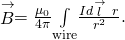As we integrate along the arc, all the contributions to the magnetic field are in the same direction (out of the page), so we can work with the magnitude of the field. The cross product turns into multiplication because the path dl and the radial direction are perpendicular. We can also substitute the arc length formula,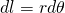: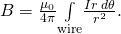The current and radius can be pulled out of the integral because they are the same regardless of where we are on the path. This leaves only the integral over the angle,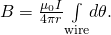The angle varies on the wire from 0 to; hence, the result is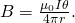Significance The direction of the magnetic field at point P is determined by the right-hand rule, as shown in the previous chapter. If there are other wires in the diagram along with the arc, and you are asked to find the net magnetic field, find each contribution from a wire or arc and add the results by superposition of vectors. Make sure to pay attention to the direction of each contribution. Also note that in a symmetric situation, like a straight or circular wire, contributions from opposite sides of point P cancel each other.

Check Your Understanding The wire loop forms a full circle of radius R and current I. What is the magnitude of the magnetic field at the center?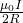### Summary

• The magnetic field created by a current-carrying wire is found by the Biot-Savart law.
• The current elementproduces a magnetic field a distance r away.

### Conceptual Questions

For calculating magnetic fields, what are the advantages and disadvantages of the Biot-Savart law?

Biot-Savart law’s advantage is that it works with any magnetic field produced by a current loop. The disadvantage is that it can take a long time.

Describe the magnetic field due to the current in two wires connected to the two terminals of a source of emf and twisted tightly around each other.

How can you decide if a wire is infinite?

If you were to go to the start of a line segment and calculate the angleto be approximately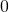, the wire can be considered infinite. This judgment is based also on the precision you need in the result.

Identical currents are carried in two circular loops; however, one loop has twice the diameter as the other loop. Compare the magnetic fields created by the loops at the center of each loop.

### Problems

A 10-A current flows through the wire shown. What is the magnitude of the magnetic field due to a 0.5-mm segment of wire as measured at (a) point A and (b) point B?Ten amps flow through a square loop where each side is 20 cm in length. At each corner of the loop is a 0.01-cm segment that connects the longer wires as shown. Calculate the magnitude of the magnetic field at the center of the loop.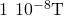What is the magnetic field at P due to the current I in the wire shown?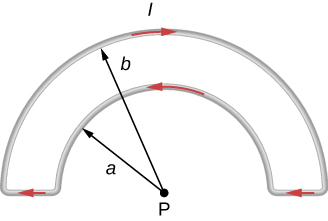The accompanying figure shows a current loop consisting of two concentric circular arcs and two perpendicular radial lines. Determine the magnetic field at point P.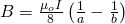out of the page

Find the magnetic field at the center C of the rectangular loop of wire shown in the accompanying figure.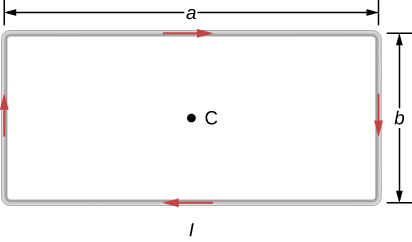Two long wires, one of which has a semicircular bend of radius R, are positioned as shown in the accompanying figure. If both wires carry a current I, how far apart must their parallel sections be so that the net magnetic field at P is zero? Does the current in the straight wire flow up or down?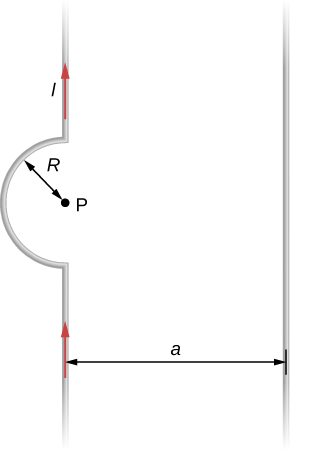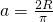; the current in the wire to the right must flow up the page.

### Glossary

Biot-Savart law
an equation giving the magnetic field at a point produced by a current-carrying wire
permeability of free space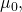measure of the ability of a material, in this case free space, to support a magnetic field